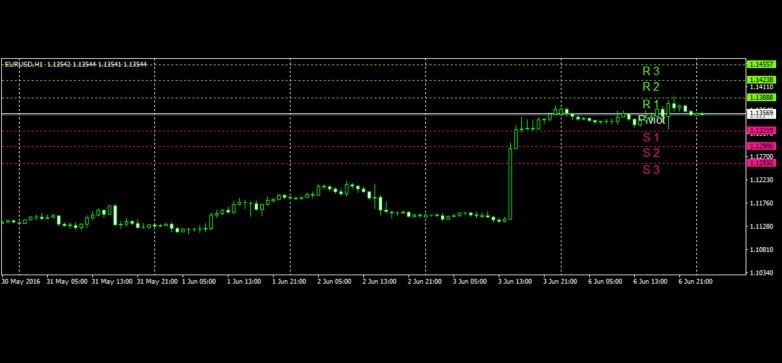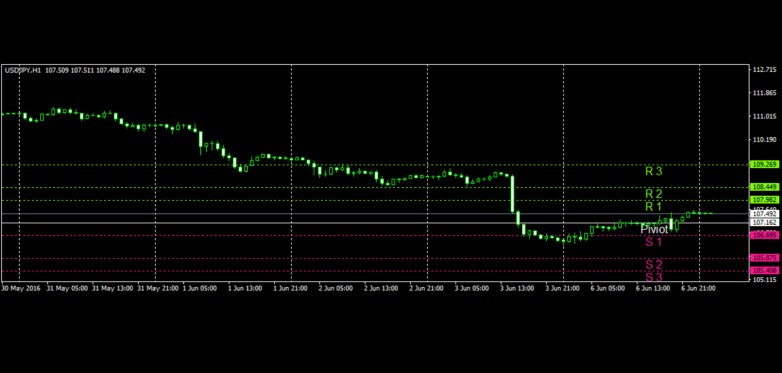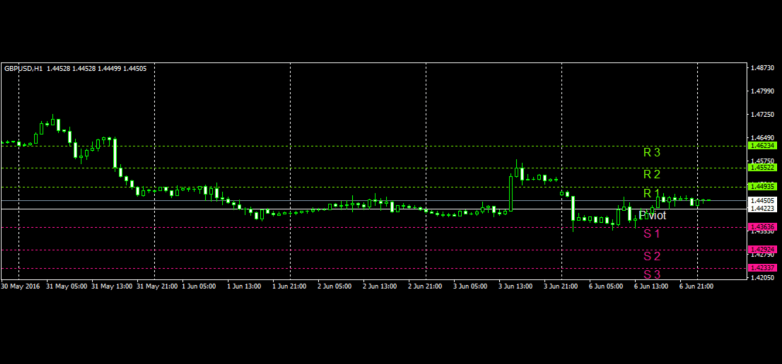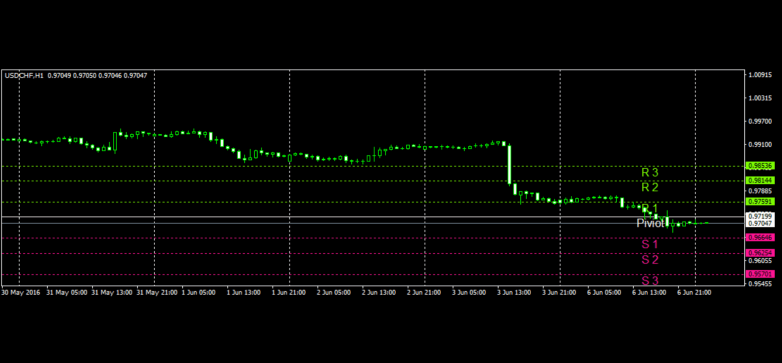HiWayFX - Analytics

## HiWayFX

452.00 9.50/10
33% of positive reviews

# Technical Analysis - 07/06/2016

## EURUSD

The EURUSD pair closed on Monday, 6th of June 2016, at 1.13562. A breakout below daily pivot which is 1.13569 will technically lead us to S1 = 1.13219, and later to S2 = 1.12900. Overextension of price will lead us to S3, which is 1.12550. A breakout above daily pivot which is 1.13569 will technically lead us to R1 = 1.13888 and later to R2 = 1.14238. Overextension of price will lead us to R3, which is 1.14557. Exponential moving average of 21 periods indicates a bullish price movement.

### Pivot Point 1 Hour

 S3 S2 S1 Daily Pivot R1 R2 R3 Classic 1.12550 1.12900 1.13219 1.13569 1.13888 1.14238 1.14557## USDJPY

The USDJPY pair closed on Monday, 6th of June 2016, at 107.518. A breakout above daily pivot which is 107.162 will technically lead us to R1 = 107.982 and later to R2 = 108.449 Overextension of price will lead us to R3, which is 109.269. A breakout below daily pivot which is 107.162 will technically lead us to S1 = 106.695, and later to S2 = 105.875. Overextension of price will lead us to S3, which is 105.408. Exponential moving average of 21 periods indicates a bearish price movement.

### Pivot Point 1 Hour

 S3 S2 S1 Daily Pivot R1 R2 R3 Classic 105.408 105.875 106.695 107.162 107.982 108.449 109.269## GBPUSD

The GBPUSD pair closed on Monday, 6th of June 2016, at 1.44436. A breakout above daily pivot which is 1.44223 will technically lead us to R1 = 1.44935 and later to R2 = 1.45522. Overextension of price will lead us to R3, which is 1.46234. A breakout below daily pivot which is 1.44223 will technically lead us to S1 = 1.43636, and later to S2 = 1.42924. Overextension of price will lead us to S3, which is 1.42337. Exponential moving average of 21 periods indicates a bearish price movement.

### Pivot Point 1 Hour

 S3 S2 S1 Daily Pivot R1 R2 R3 Classic 1.42337 1.42924 1.43636 1.44223 1.44935 1.45522 1.46234## USDCHF

The USDCHF pair closed on Monday, 6th of June 2016, at 0.97040 A breakout below daily pivot which is 0.97199 will technically lead us to S1 = 0.96646 and later to S2 = 0.96254. Overextension of price will lead us to S3, which is 1.95701. A breakout above daily pivot which is 0.97199 will technically lead us to R1 = 0.97591, and later to R2 = 0.98144. Overextension of price will lead us to R3, which is 0.98536. Exponential moving average of 21 periods indicates a bearish price movement.

### Pivot Point 1 Hour

 S3 S2 S1 Daily Pivot R1 R2 R3 Classic 1.95701 0.96254 0.96646 0.97199 0.97591 0.98144 0.98536